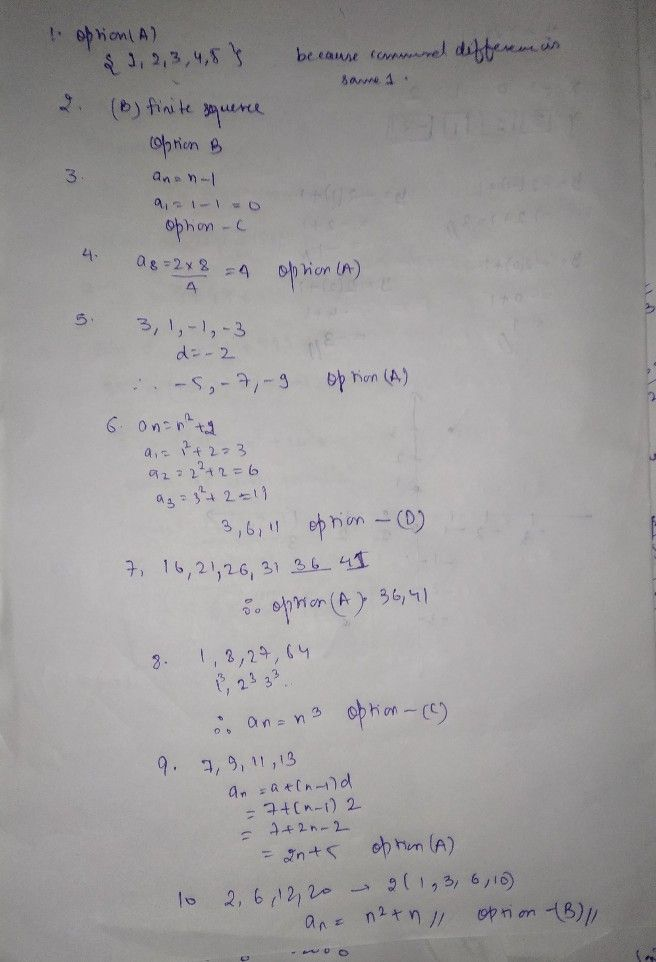Symbol
Problem1. Which of the following set of numbers is an example of a A. B. $1.2$ $24$ 3, 4, 5} C. (1,4, $sc9$ $onc07$ $9.16.27\right)$ $24.6\right)$ 8, 16, 31) D. (1. $2.111s9$ function whose domain includes only the first n positive integers A. Infinite Sequence C. Infinite Series B. Finite Sequence D. Finite scrics 3. What is the value of the first term of the sequence a = n-1? A. -1 B. 1 C. 0 D. 2 4. Which of the following numbers is the 8" term of sequence a, = D. 16 A. 4 B. 8 C. 12 5. Using the pattem 3, 1, -1, -3 what are the next three terms of the sequence? A. -5, -7, -9 CD. . -5, Dur B.-1, 1, 3 -3, --91, , 7 1 6. What are the first three terms of the sequence with the nth term $a=n^{2}+27$ C. 3, 6, 8 ep A. 3, 6, 15 D. 3, 6, 11 B. 3, 6, 10 7. What are the next two numbers that will complete the terms of the sequence 16, $21.26.31$ A. 36, 41 $41.46$ $40$ $4$ B. $4245$ 8. Find the general rule of the sequence $c$ $1.8.27.64^{0}$ $c$ $0$ $9==2n-1$ $a_{n}=n^{3}$ $a_{n}=2n^{2}$ $A$ $θ=2$ $B.a_{n}=n$ 9. Which of the following equation is the n" term of the sequence $7.y,11$ 13, 15, ...? $a=2n+5|$ C. $a_{n}=2n-5$ $a_{3}=2n-3$ BA. . $9=2n+3$ D. 10. What is the nth tem of the sequence $26,12.20$ $c$ $0$ $2$ $0n=2n^{2}+1$ $8n=2n^{2}-1$ $A$ $B=\pi ^{2}-$ $B.Bn=x+n$
10th-13th grade
Other
Search count: 107
SolutionQanda teacher - RakeshRajpls rateStudent
Thank you!Qanda teacher - RakeshRaj
wlcm
pls rate it